# Li-Ion Diffusion Coefficients in cathode materials¶

In this tutorial we will compute the diffusion coefficients of Lithium atoms in a Li0.4S cathode by means of molecular dynamics simulations with the ReaxFF engine.

The systems and workflows presented here are originally described in the publication ReaxFF molecular dynamics simulations on lithiated sulfur cathode materials Phys. Chem. Chem. Phys. 17, 3383-3393 (2015).

The tutorial consists of the following steps:

• Importing a CIF file
• Manipulating the structure (e.g. inserting particles) and equilibrating the system
• Creating an amorphous structure by simulated annealing
• Calculating diffusion coefficients from MD trajectories

If you wish, you can download the already-relaxed amorphous Li0.4S structure by clicking here and skip the simulated annealing and equilibration calculations.

## Importing the Sulfur(α) crystal structure¶

1. Open a new AMSinput window

To speed up the calculations, we will use a smaller system than the one described in the publication. The crystal structure can be directly imported from a CIF file:

2. In AMSinput: File → Import Coordinates
3. Select the CIF file you just downloaded using the file dialog window

## Generating the Li0.4S system¶

We first need an xyz file of a single Lithium atom:

1. Open a new AMSinput: SCM → New Input
2. Draw a single Li atom
3. File → Export coordinates → .xyz and give it the name “Li.xyz”
4. Close the AMSinput window without saving

We use the builder functionality of AMSinput to randomly insert 51 Li-atoms into our Sulfur system.

A better way of building the Li0.4S system is to use Gran Canonical Monte Carlo (GCMC). See also the Battery discharge voltage profiles using Grand Canonical Monte Carlo tutorial.

1. Click on Edit → Builder
2. Next to “Fill box with”, set the number to 51
3. Click on the folder and select the “Li.xyz” you just made
4. Set Distance to 1.0 Å
5. Click on Generate Molecules
6. Click on CloseWe now need to relax the geometry by running a geometry optimization including lattice relaxation:

1. In the main panel, select Task → Geometry Optimization
2. Click on the folder next to Force Field and select LiS.ff
3. Details → Geometry Optimization
4. Tick the Optimize lattice checkbox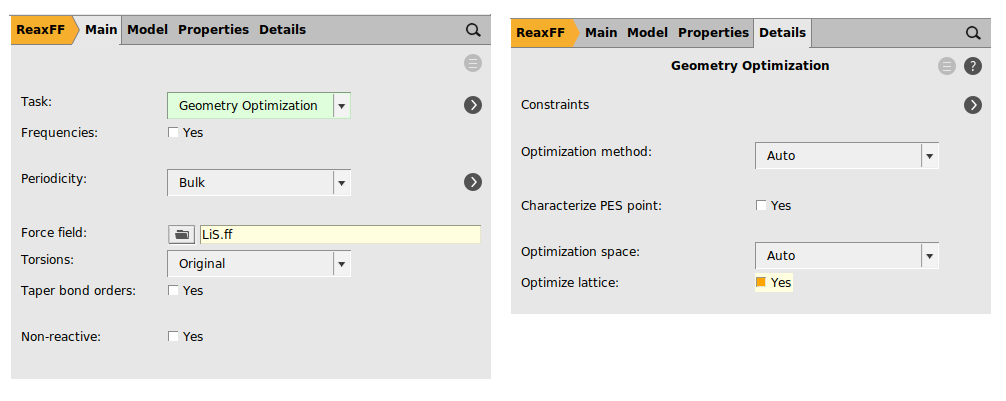We are now ready to run the Li0.4S relaxation calculation:

1. File → Save As… and give it an appropriate name (e.g. “LiS_optimization”)
2. File → Run
3. Click yes when asked if the structure in AMSinput should be updated

The volume of the unit cell should have increased significantly during the optimization (from about 3300 Å3 to about 4400 Å3):

1. Open AMSmovie by clicking on SCM → Movie
2. In AMSmovie, click on Graph → Cell volume
3. Click on play to see the movie of the geometry optimization## Creating the amorphous systems by simulated annealing¶

Amorphous systems can be created with a Molecular Dynamics simulation by slowly heating up the system followed by a rapid cool-down.

As in the publication, we will anneal up to 1600 K followed by a rapid cool-down to room temperature. In order to speed up the calculation, only 30000 steps are calculated here.

1. In the main panel, select Task → Molecular Dynamics
2. Click on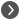next to Task: Molecular Dynamics to go to the MD details
3. Set the number of steps to 30000We will now set up the following temperature profile:

1. From start until step 5000: T = 300 K (constant)
2. From step 5000 to step 25000: heating up from 300 K to 1600 K
3. From step 25000 to step 30000: cooling down from 1600 K to 300 K

For more details on temperatures and pressure regimes, see the AMS manual on MD.

1. Click onnext to Thermostat to go to the thermostat details
3. Select Thermostat → Berensden
4. In Temperature(s), set the values 300 300 1600 300
5. In Duration(s), set the values 5000 20000 5000
6. Set the damping constant to 100 fsWe are now ready to run the Li0.4S simulated annealing calculation:

1. File → Save As… and give it an appropriate name (e.g. “LiS_simulated_annealing”)
2. File → Run
3. Click yes when asked if the structure in AMSinput should be updated

In AMSmovie you can follow the progress of the MD simulation, and you’ll be able to see the three temperature regimes:

1. Open AMSmovie by clicking on SCM → Movie
2. In AMSmovie, click on MD Properties → Temperature
3. Click on play to see the movie of the MD simulation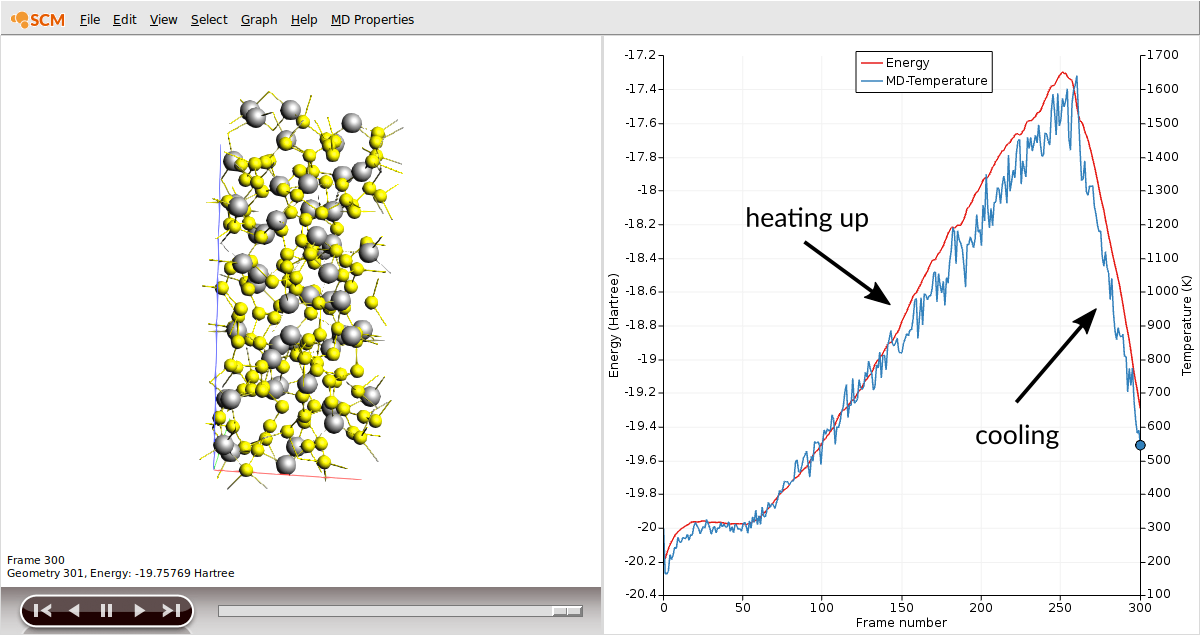We now need to relax the geometry of our new amorphous system by running a geometry optimization including lattice relaxation (as we did before):

1. In the main panel, select Task → Geometry Optimization
2. Details → Geometry Optimization
3. Tick the Optimize lattice checkbox
4. File → Save As… and give it an appropriate name (e.g. “LiS_amorphous_optimization”)
5. Run the calculation
6. Click yes when asked if the structure in AMSinput should be updated

## Calculating the diffusion coefficients¶

We are now ready to run the final MD simulation to compute the Lithium diffusion coefficient at T=1600 K.

AMS calculates the diffusion coefficient by integration of the velocity autocorrelation function:

$D = D = \frac{1}{3} \int_{t=0}^{t=t_{max}} \langle \textbf{v}(0) \cdot \textbf{v}(t) \rangle \rm{d}t$

To compute $$D$$ accurately in this way, it is necessary to frequently write the atomic velocities to the trajectory file.

We will use a minimal proof-of-principle setup of 10000 equilibration steps followed by only 100000 production steps:

1. In the main panel, select Task → Molecular Dynamics
2. Click onnext to Task: Molecular Dynamics to go to the MD details
3. Set the number of steps to 110000
4. Set the Sample frequency to 5 (this writes both the atomic positions and velocities to disk every 5 steps)
5. Click onnext to Thermostat to go to the thermostat details
6. Set the Temperature to 1600 K
7. Clear the Duration field
8. Set the damping constant to 100 fs

We are now ready to run the calculation:

1. File → Save As… and give it an appropriate name (e.g. “LiS_MD_1600K”)
2. File → Run
3. You can follow the progress of the calculation in AMSMovie and AMStail (SCM → Logfile)

After the calculation is finished, we can obtain the Li diffusion coefficient by computing the velocity autocorrelation function for the Li atoms:

1. In AMSMovie select MD Properties → Autocorrelation function
2. In Steps set 2000 - 22001
3. Select Properties → Diffusion Coefficient (D)
4. In the visualization area, select one of the Li atoms, and then Select → Select atoms of the same type
5. With all Lithium atoms selected, click on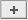next to Atoms: in the Autocorrelation Function panel
6. Click on Generate ACF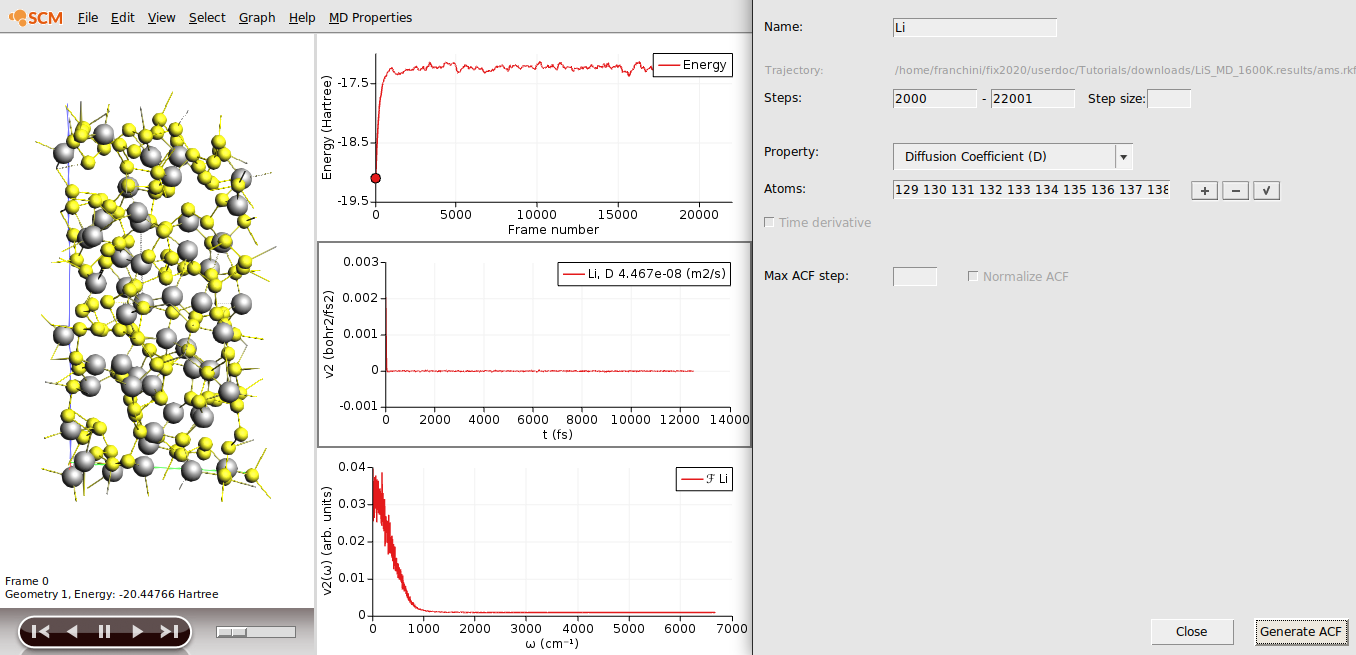The value of the Diffusion Coefficient D for the Li atoms is $$4.5 \times 10^{-8}$$ m2/s (since we are not running a very long MD simulations, you might obtain a slightly different value).

Calculating the diffusion coefficient at 300K would require a very long trajectory. However, it is possible to provide an upper bound to the Li diffusion by means of extrapolation from elevated temperatures using the Arrhenius equation:

$D(T) = D_0 \exp{(-E_a / k_{B}T)}$
$\ln{D(T)} = \ln{D_0} - \frac{E_a}{k_{B}}\cdot\frac{1}{T}$

where $$D_0$$ is the pre-exponential factor, $$E_a$$ is the activation energy, $$k_B$$ is the Boltzmann constant, and $$T$$ is the temperature. The activation energy and pre-exponential factors can then be obtained from an Arrhenius plot of $$\ln{(D(T))}$$ against $$1/T$$. In order to extrapolate the diffusion coefficients for Li0.4S we calculate trajectories for at least four different temperatures (600 K, 800 K, 1200 K, 1600 K) for each system. One can then extrapolate the diffusion coefficient to lower temperature.